# JS中this关键字的指的是什么

this是Javascript语言的一个关键字。

function test(){
this.x = 1;
}

function test(){
this.x = 1;
}
test(); // 1

var x = 1;
function test(){
}
test(); // 1

var x = 1;
function test(){
this.x = 0;
}
test();

function test(){
}
var o = {};
o.x = 1;
o.m = test;
o.m(); // 1

function test(){
this.x = 1;
}
var o = new test();

var x = 2;
function test(){
this.x = 1;
}
var o = new test();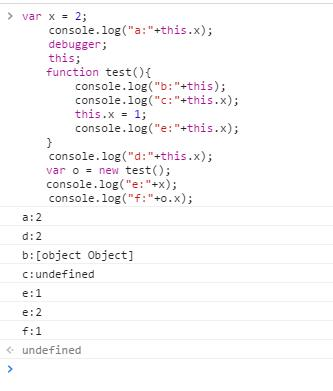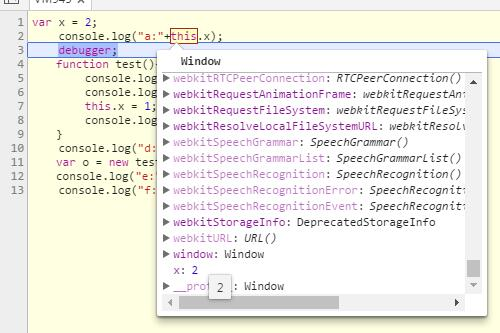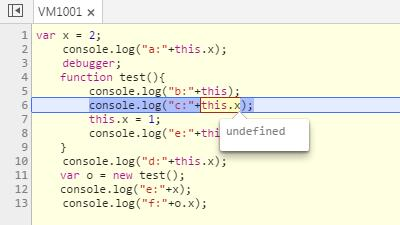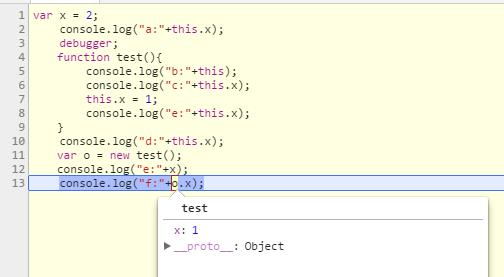apply()是函数对象的一个方法，它的作用是改变函数的调用对象，它的第一个参数就表示改变后的调用这个函数的对象。因此，this指的就是这第一个参数。

var x = 0;
function test(){
}
var o={};
o.x = 1;
o.m = test;
o.m.apply(); //0

apply()的参数为空时，默认调用全局对象。因此，这时的运行结果为0，证明this指的是全局对象。

o.m.apply(o); //1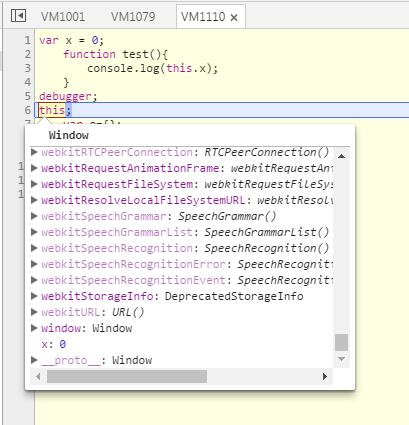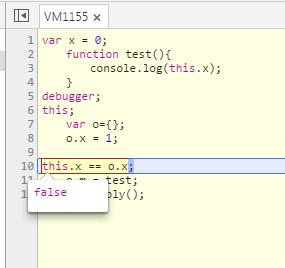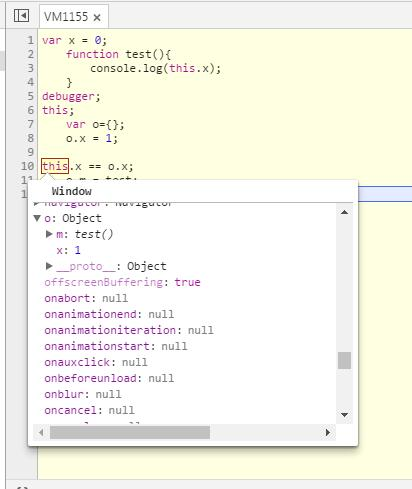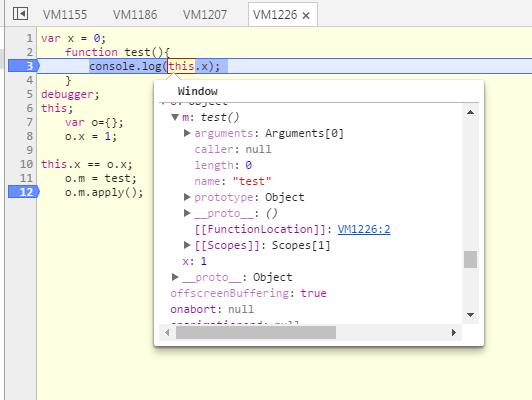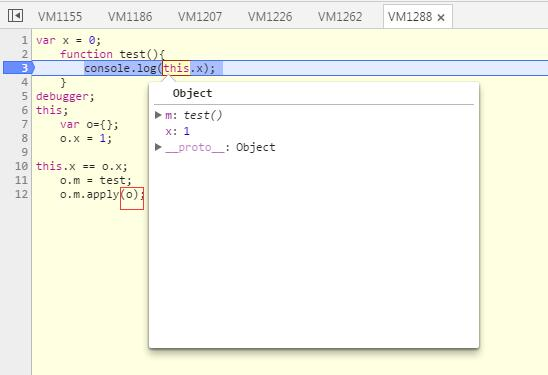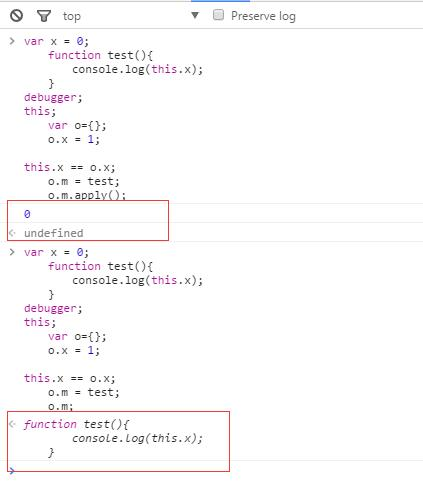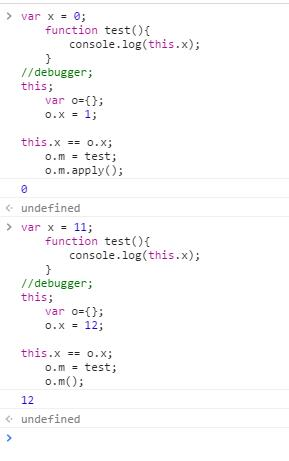## 本文地址: http://blog.csdn.net/aerchi/article/details/68490788

©️2019 CSDN 皮肤主题: 编程工作室 设计师: CSDN官方博客